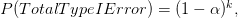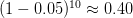# What is the multiple testing problem? How does FigPii deal with it?

Short answer: FigPii uses Bayesian statistics to avoid the "multiple testing" problem.

According to Michael Frasco:

Bayesian A/B testing accomplishes this without sacrificing reliability by controlling the magnitude of our bad decisions instead of the false positive rate.

A brief explanation of the "multiple testing" problem When conducting a two-tailed test that compares two conversion rates of the control P and the conversion rate for the variation (P), your hypothesis would be:

Null hypothesis: H₀ : P₁ = P

Alternative hypothesis: H₀ : P₁  P₂

Your goal in conducting the AB test is to reject the null (H0) hypothesis that both rates are equal. You never accept the null hypothesis. If your test does not result in a winner, it means that you do not have enough evidence/data to reject the null hypothesis. If the null hypothesis is true, so the two rates are equal, then you do not reject H0, and your decision is correct. The same applies when your test has a winner, which means that the null hypothesis (H0) is false and you reject it correctly. However, when you reject a true null hypothesis (H0), you make a type I error (false positive). Similarly when the null hypothesis (H0) is false, but you fail to reject, then you make a type II error (false negative). With many comparisons, the probability of discovering AT LEAST ONE false significant result, i.e., incorrectly rejecting a null hypothesis, increases according to a formula:Where k is the number of variations in a test. So for a test that contains 10 different variations and a significance level of 5% (k equal 10 and alpha 0.05), the overall type I error increases too.This means that you have a 40% chance of finding a false negative: assuming that at least one of your variations is better [or worse] than the control when in fact it is not. So, with ten variations your test has a 50% chance of getting a significant false result. You can read a more detailed description of the problem here.